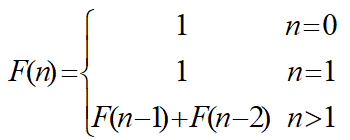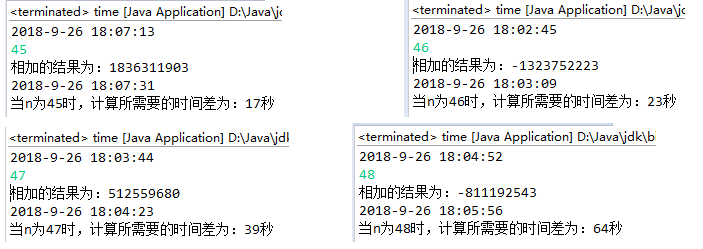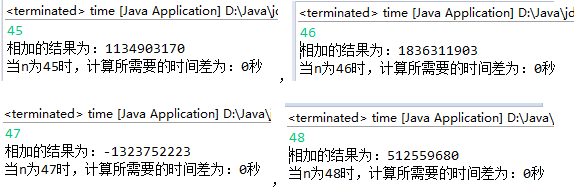• 主要为大家详细介绍了Java递归实现斐波那契数列，具有一定的参考价值，感兴趣的小伙伴们可以参考一下
• 主要为大家详细介绍了JAVA递归与非递归实现斐波那契数列，具有一定的参考价值，感兴趣的小伙伴们可以参考一下
• 实现代码如下: public static int factorial(int n){ if (n <= 1){ return 1; } return factorial(n-1) + factorial(n-2); } 测试代码如下: System.out.println(factorial(40)); ...测...
实现代码如下:
public static int factorial(int n){
if (n <= 1){
return 1;
}
return factorial(n-1) + factorial(n-2);
}

测试代码如下:
System.out.println(factorial(40));

测试结果如下:
165580141

展开全文• 斐波那契（Leonardoda Fibonacci）以兔子繁殖为例子而引入，故又称为“兔子数列”，指的是这样一个数列：1、1、2、3、5、8、13、21、34、……在数学上，斐波纳契数列以如下被以 递归的方法定义：F(0)=0，F(1)=1, F(n...
前言：
斐波那契数列（Fibonacci sequence），又称 黄金分割数列、因数学家列昂纳多·斐波那契（Leonardoda Fibonacci）以兔子繁殖为例子而引入，故又称为“ 兔子数列”，指的是这样一个数列：1、1、2、3、5、8、13、21、34、……在数学上，斐波纳契数列以如下被以 递归的方法定义：F(0)=0，F(1)=1, F(n)=F(n-1)+F(n-2)（n>=2，n∈N*）在现代物理、准晶体结构、化学等领域，斐波纳契数列都有直接的应用，为此，美国数学会从1963起出版了以《斐波纳契数列季刊》为名的一份数学杂志，用于专门刊载这方面的研究成果。
代码很简单，实例如下：
public static int fibonacciSequence(int i){
if(i <= 1){
return i;
}
return fibonacciSequence(i-1)+fibonacciSequence(i-2);
}
public static void main(String[] args) {
System.out.println("请输入要计算第多少位数字:");
Scanner scanner = new Scanner(System.in);
int next = scanner.nextInt();
for (int i = 0; i < next; i++) {
System.out.println(fibonacciSequence(i));
}

}


展开全文web
• 递归法计算Fibonacci数列： 它可以递归地定义为： 第n个Fibonacci数列递归地计算如下： int fibonacci(int n)  {  if (n &lt;= 1) return 1;  return fibonacci(n-1)+fibonacci(n-2);  } 以下这...
递归法计算Fibonacci数列：
它可以递归地定义为：第n个Fibonacci数列可递归地计算如下：
int fibonacci(int n)
{
if (n <= 1) return 1;
return fibonacci(n-1)+fibonacci(n-2);
}
以下这个源代码可以计算出递归法实现Fibonacci数列时，n为45、46、47、48时所需的时间。
import java.text.DateFormat;
import java.util.Date;
import java.util.Scanner;
public class time {
public static void main(String[] args) {

Date date=new Date();
DateFormat df=DateFormat.getDateTimeInstance();

System.out.println(df.format(date));
Scanner in=new Scanner(System.in);
int n=in.nextInt();

time a=new time();
a.Fib(n);
System.out.println("相加的结果为："+Fib(n));

Date date1=new Date();
System.out.println(df.format(date1));
long time=(date1.getTime()-date.getTime())/1000;
System.out.println("当n为"+n+"时，计算所需要的时间差为："+time+"秒");
}
public static int Fib(int n){
if(n<=1) return 1;
return Fib(n-1)+Fib(n-2);
}
}可以看到，递归法所需要的时间还是很久的，因为计算F(n)时，需首先计算F（n-1）和F(n-2)
，而在计算F（n-1）时已经算过F(n-2)了，但是递归算法看不到这点，所以产生这么多假发，所需时间也就多了，效率就下降了。
尾递归：
尾递归比递归函数效率高太多了，尾递归就是把当前的运算结果（或路径）放在参数里传给下层函数。而不是把下层函数的运算结果用来本次的计算。尾递归是极其重要的，不用尾递归，函数的堆栈耗用难以估量，需要保存很多中间函数的堆栈，而递归每一次计算出来的部分结果，在下一次循环时还需要再计算一遍。
import java.text.DateFormat;
import java.util.Date;
import java.util.Scanner;
public class time {
public static void main(String[] args) {

Date date=new Date();
DateFormat df=DateFormat.getDateTimeInstance();
Scanner in=new Scanner(System.in);
int n=in.nextInt();

time a=new time();
a.Fib(n);
System.out.println("相加的结果为："+Fib(n));
Date date1=new Date();
long time=(date1.getTime()-date.getTime())/1000;
System.out.println("当n为"+n+"时，计算所需要的时间差为："+time+"秒");
}
public static int Fib(int n){
if(n<2) return n;
return Fibo(n,1,1,3);
}
public static int Fibo(int n,int r1,int r2,int begin){
if(n==begin) return r1+r2;
return Fibo(n,r2,r1+r2,++begin);
}
}展开全文• 斐波那契数列：指的是这样一个数列 0, 1, 1, 2, 3, 5, 8, 13, 21, 34, 55, 89, 144, 233，377，610，987，1597，2584，4181，6765，10946，17711，28657，46368…… 注意：此时我们需要注意的是该数列第一项是0，第...
斐波那契数列：指的是这样一个数列 0, 1, 1, 2, 3, 5, 8, 13, 21, 34, 55, 89, 144, 233，377，610，987，1597，2584，4181，6765，10946，17711，28657，46368…… 注意：此时我们需要注意的是该数列第一项是0，第二项是1，接下来的每一项则是前两项之和 思路：这个题我们需要用到递归的方法，首先我们需要先解决数列前两项已知的项，我们首先定义一个静态函数feibonaqie（）， 在函数当中，我们先利用一个if语句去判断前两项，如果发现是前两项，则直接返回0和1；否则我们返回的值就等于前两项之和，于是我们就可以使用递归函数让我们返回的值为前两项之和。
总结：此类型的题都需要我们熟练使用递归的方法，如果对递归不熟悉的同学可以点击下面这个链接进入我的另外一篇博客对递归方法进行学习。递归学习
import java.util.Scanner;
public class Fibonaqie {
public static long feibonaqie(long number)
{
if (number==0||number==1)
{
return number;
}
else
{
return feibonaqie(number-1)+feibonaqie(number-2);
}
}
public static void main(String args[])
{
Scanner input = new Scanner(System.in);
System.out.println("请输入需要计算第几项的值：");
long number = input.nextLong();
System.out.println("第"+number+"项的斐波那锲值为："+feibonaqie(number));
}
}

展开全文算法
• 斐波那契数列Fibonacci sequence），又称黄金分割数列、因数学家列昂纳多·斐波那契（Leonardoda Fibonacci）以兔子繁殖为例子而引入，故又称为“兔子数列”，指的是这样一个数列：1、1、2、3、5、8、13、21、34、...
• 斐波那契数列指的是这样一个数列 1, 1, 2, 3, 5, 8, 13, 21, 34, 55, 89, 144, 233，377，610，987，1597，2584...//递归斐波那契数列的第n个数值 //递归就是自己调用自己 class Evaluate//求值类 { private int a1
• 斐波那契数列 1 1 2 3 5 8 13 21 34 一个数等于前两个数之和 计算斐波那契数列第n个值并打印出来 public class practice { ...//递归斐波那契数列 public int method (int n) { if(n==1) { retu.算法
• import java.util.Scanner; public class Test36{ public static void main(String[] args) { Scanner sc=new Scanner(System.in); int n=sc.nextInt(); int sum=feibo(n);...
• public static int fibonacciSequence(int i) { if (i <= 1) { return i; } return fibonacciSequence(i - 1) + fibonacciSequence(i - 2); } public static void main(String[] args) { System.out....算法
• 代码如下： ...public class Fibonacci { //递归 public static long f_recursion(int n){ return n<=1? 1 : f_recursion(n-2)+f_recursion(n-1); } //非递归 public static long f(int n)...
• 输入你要求的第几个斐波那契数列的值,打印出对应的值(利用递归) import java.util.Scanner; class TestFibonacci{ public static void main(String[] args){ Scanner sc = new Scanner(System.in); System.out....
• public class Fibonacci { public static void main(String[] args) { Scanner input = new Scanner(System.in); System.out.println("输出几个斐波那契数？"); int n=input.nextInt(); System.out.println(fib...
• 斐波那契数列Fibonacci sequence），又称黄金分割数列，因数学家莱昂纳多·斐波那契（Leonardo Fibonacci）以兔子繁殖为例子而引入，故又称为“兔子数列”，指的是这样一个数列：0、1、1、2、3、5、8、13、21、34...1024程序员节
• public class Fibonacci { public static void main(String[] args) { Scanner sc = new Scanner(System.in); int n = sc.nextInt(); int fn = fib(n);...斐波那契数列的第n项为：" + fn); } ...
• 递归实现斐波那契数列 1、1、2、3、5、8、13、21、... F(1)=1, f(2)=1 F(N)=F(N-1)+F(N-2) 分析下 我们发现的规律是 F(N)=F(N-1)+F(N-2) 而且有结束 F(1)=1, f(2)=1 我们上代码： 1 2 3 ...
• //Fibonacci数列：1，1,2,3,5,8，... //求该数列第n个数的值。 //数列满足递归公式：F1=1，F2+=1,Fn=Fn-1 + Fn-2 public class Fibonacci { public static void main(String[] args){ System...递推算法
• 递归分解为：直接量+小规模子问题； 也可分解为：多个小规模子问题； 1,1,2,3,5,8,13 当n=1,2时 f(n)=1; 当n&gt;3时 f(n)=f(n-1)+f(n-2) public class Main{ public static void main(String[] args){ ...
• /** *create Date:2016-12-23 *modified Date:2016-12-23 ...*Description:斐波那契数列 **/ public class Shulie{ public static long digui(int n,int sum,int before,int before1){ if
• /** @Description @author jhonjens Email:jhonjens@qq.com @version ...@date 2019年11月14日下午2:39:34 ...public static void main(String[] args) { // 斐波那契数列 // 输入一个数据n，计算斐波那契...
• import java.util.Scanner; public class Fibonacci { public static void main(String[] args) { // TODO Auto-generated method stub System.out.println("请问需要输出斐波那契数列的前多少项？"); Sc...
• 斐波那契数列：0,1,1,2,3,5,8,13,21…第三个数始终为前两个数相加的和 public class Solution { public int Fibonacci(int n) { if(n == 0) { return 0; } if(n == 1) { return 1; ...
• 可以计算从1到你输入的那个数之间的斐波那契数列
• public static int fib... //递归斐波那契数列的第 N 项 //1 1 2 3 5 8 if (n == 1 || n ==2) { return 1; } return fib(n-1) + fib(n-2); } public static void main(String[] args) { System.out.printlnleetcode 开发语言
• 程序调用自身的编程技巧称为递归（ recursion）。递归做为一种算法在程序设计语言中广泛应用。 一个过程或函数在其定义或说明中有直接或间接调用自身的一种方法，它通常把一个大型复杂的问题层层转化为一个与原问题...函数 recursion 算法 对象
• 斐波那契数列 *以兔子繁殖为例子而引入，指的是这样一个数列：0、1、1、2、3、5、8、13、21、34、…… * 在数学逻辑上以递归方法定义
• C/C++ 递归斐波那契数列【简单易懂，代码可以直接运行】 老生常谈了，C/C++/C#/Java/python都有这种题 递归途径类似于下面这种，区别就是这里有一个递归斐波那契数列有两个递归，就这一点区别！ 请使用递归的...c++ python 算法
• 通过java递归法解斐波那契数列，有具体实现代码及递归思想的简单介绍算法 字符串...

# java递归打印斐波那契数列java 订阅